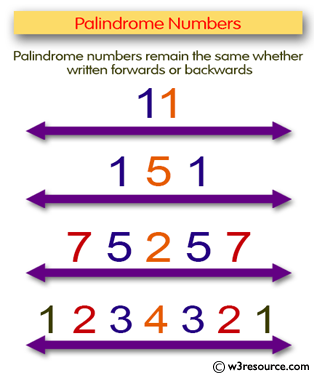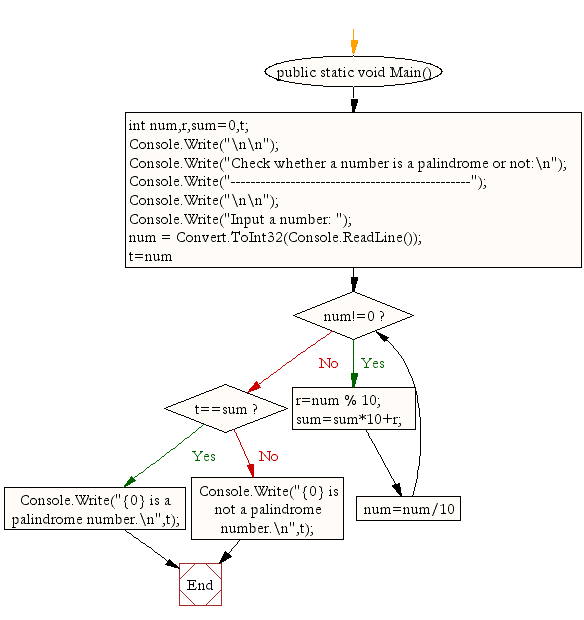﻿ C# - Check whether a number is a palindrome or not# C# Sharp Exercises: Check whether a number is a palindrome or not

## C# Sharp For Loop: Exercise-38 with Solution

Write a program in C# Sharp to check whether a number is a palindrome or not.

Pictorial Presentation:Sample Solution:

C# Sharp Code:

``````using System;
public class Exercise38
{
public static void Main()
{
int num,r,sum=0,t;

Console.Write("\n\n");
Console.Write("Check whether a number is a palindrome or not:\n");
Console.Write("------------------------------------------------");
Console.Write("\n\n");

Console.Write("Input a number: ");

for(t=num;num!=0;num=num/10){
r=num % 10;
sum=sum*10+r;
}
if(t==sum)
Console.Write("{0} is a palindrome number.\n",t);
else
Console.Write("{0} is not a palindrome number.\n",t);
}
}
```
```

Sample Output:

```Check whether a number is a palindrome or not:
------------------------------------------------
Input a number: 8
8 is a palindrome number. ```

Flowchart:C# Sharp Code Editor:

Contribute your code and comments through Disqus.

What is the difficulty level of this exercise?

Test your Programming skills with w3resource's quiz.

﻿